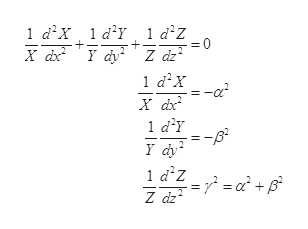# Suppose that we want to solve Laplace’s equation inside a hollow rectangular box, with sides of length a, b and c in the x, y and z directions, respectively. Let us set up the axes so that the origin is at one corner of the box, so that the faces are located at x = 0 and x = a; at y = 0 and y = b; and at z = 0 and z = c. Suppose that the faces are all held at zero potential, except for the face atz=c,onwhichthepotentialisspecifiedtobeV(x,y,c)=V0 =const.a) Find the electrostatic potential V at a generic point inside the box.b) Find the expression for the electrostatic potential evaluated at the center of the box, i.e. deter-mine V (a/2, b/2, c/2). Simplify your answer as much as you can!c) Suppose now that a = b = c, i.e. the box is a cube. Give a simple argument which gives theexact (and simple) expression for the potential at the center of the cube. (No calculations are asked here. Use physics, wave your hands, etc. and say “the answer is such and such because ...”)

Question

Suppose that we want to solve Laplace’s equation inside a hollow rectangular box, with sides of length a, b and c in the x, y and z directions, respectively. Let us set up the axes so that the origin is at one corner of the box, so that the faces are located at x = 0 and x = a; at y = 0 and y = b; and at z = 0 and z = c. Suppose that the faces are all held at zero potential, except for the face atz=c,onwhichthepotentialisspecifiedtobeV(x,y,c)=V0 =const.

a) Find the electrostatic potential V at a generic point inside the box.
b) Find the expression for the electrostatic potential evaluated at the center of the box, i.e. deter-

mine V (a/2, b/2, c/2). Simplify your answer as much as you can!

c) Suppose now that a = b = c, i.e. the box is a cube. Give a simple argument which gives theexact (and simple) expression for the potential at the center of the cube. (No calculations are asked here. Use physics, wave your hands, etc. and say “the answer is such and such because ...”)

check_circleExpert Solution
Step 1

(a) Write the Laplace’s equation

Step 2

Using the method of separation of variables

Step 3

Substituting the above equation i...help_outlineImage Transcriptionclose1 а'x, 1а'r 1 d'z = 0 1 d2x -a2 1 d'Y Y фу- 1 d'z = 7 = a + Z dz fullscreen

### Want to see the full answer?

See Solution

#### Want to see this answer and more?

Solutions are written by subject experts who are available 24/7. Questions are typically answered within 1 hour*

See Solution
*Response times may vary by subject and question
Tagged in

### Science